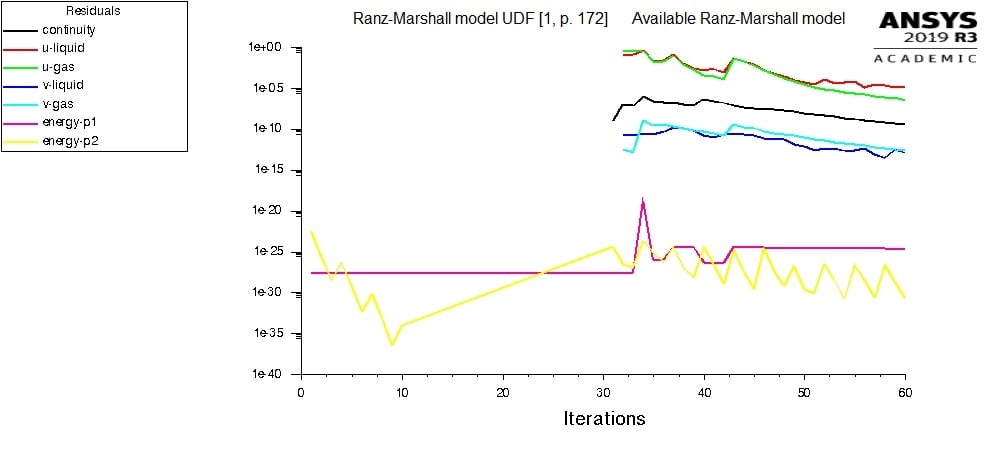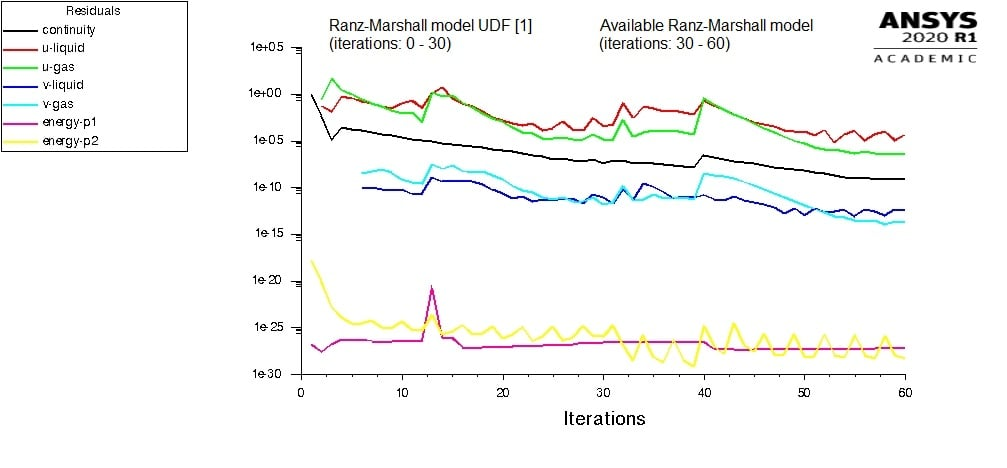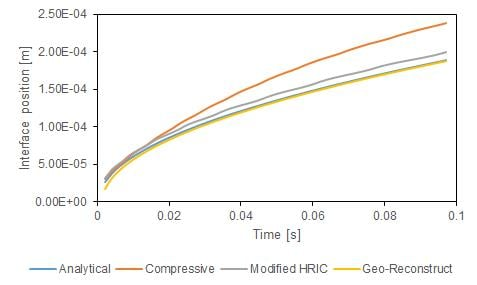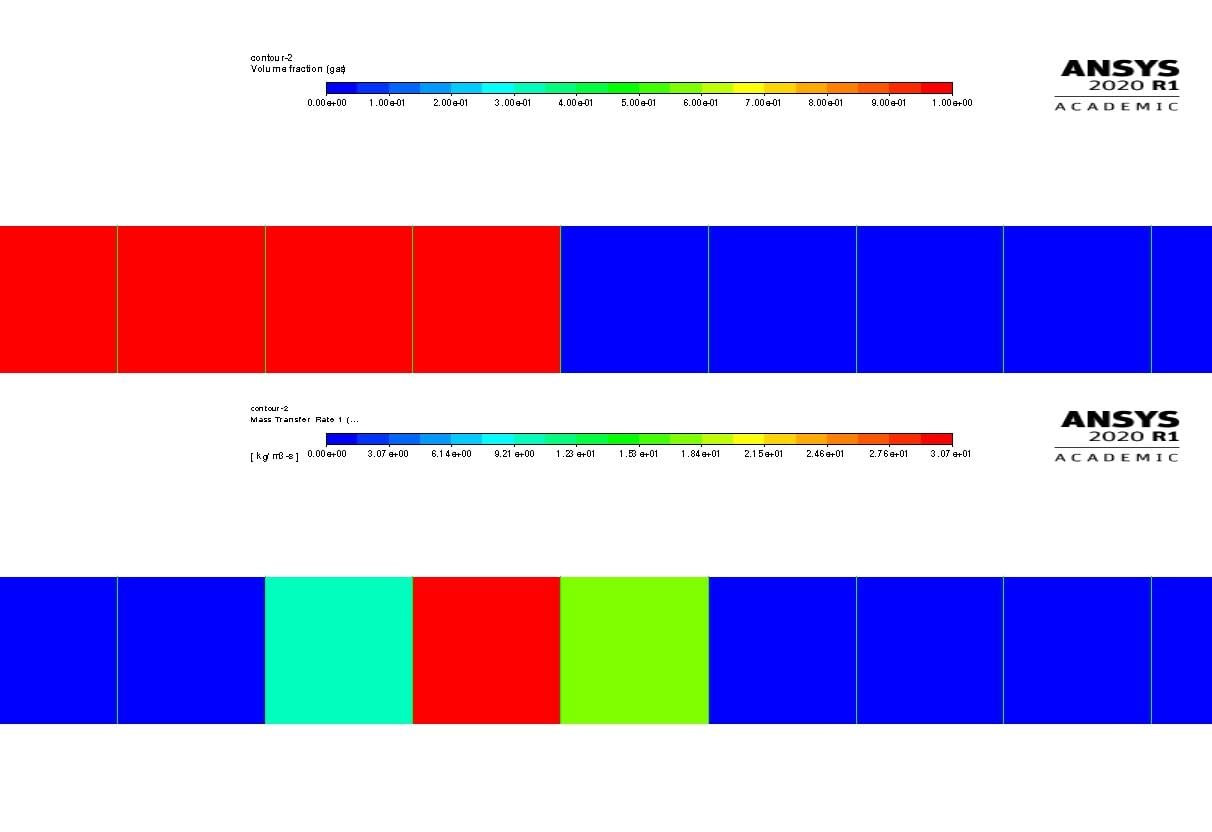# Why only energy equations are solved when HTC is defined via UDF in Eulerian Multiphase model?

Hi,

my goal is to model interphase heat transfer inside "Two-Resistance" model using user-defined volumetric heat transfer coefficient (HTC) at "To-Phase" in Eulerian Multiphase solver implemented in academic version of ANSYS Fluent 2019 R3.

Incorporation of user-defined volumetric HTC results in solution of only the energy equations, as shown in the attached figure. Here, the code for "Ranz-Marshall" model available in [1, p. 172] is used.

However, if "Ranz-Marshall" model already available in the software is used, all the conservation equations are being solved, as denoted in the figure.

The question is: "What is missing and causing solution of only energy equations?"

Best regards,

Alen

Reference
 ANSYS, Inc., ANSYS Fluent Customization Manual, Release 2019 R2, 2019.• edited February 27
How are you defining the HTC? Are you switching any eqs off?
• edited February 27

Dear Mr. Amine,

thank you for response.

The volumetric HTC is defined as [1, p. 612]:

h_pq = k_q * Nu_p / d_p,

where "h_pq" is the volumetric HTC, "k_q" is the thermal conductivity of the continuous phase, "Nu_p" is Nusselt number and "d_p" is the diameter of the dispersed phase.

The value returned by "DEFINE_EXCHANGE_PROPERTY" macro is:

val = h_pq * A_int,

where "A_int" is the interfacial area.

The computation of interphase heat transfer on the side of the dispersed phase ("water-vapor", "To-Phase") in "Two-Resistance" model is conducted using the code available in UDF manual [2, p. 172] that reads:

"#include "udf.h"
#define PR_NUMBER(cp,mu,k) ((cp)*(mu)/(k))
#define IP_HEAT_COEFF(vof,k,nu,d) ((vof)*6.*(k)*(Nu)/(d)/(d))

{
real h;
real d = C_PHASE_DIAMETER(c,tj);
real k = C_K_L(c,ti);
real NV_VEC(v), vel, Re, Pr, Nu;
NV_DD(v,=,C_U(c,tj),C_V(c,tj),C_W(c,tj),-,C_U(c,ti),C_V(c,ti),C_W(c,ti));
vel = NV_MAG(v);
Re = RE_NUMBER(C_R(c,ti),vel,d,C_MU_L(c,ti));
Pr = PR_NUMBER (C_CP(c,ti),C_MU_L(c,ti),k);
Nu = 2. + 0.6*sqrt(Re)*pow(Pr,1./3.);
h = IP_HEAT_COEFF(C_VOF(c,tj),k,Nu,d);
return h;
}

DEFINE_EXCHANGE_PROPERTY(heat_udf, c, t, i, j)
{
real val;
val = heat_ranz_marshall(c,ti, tj);
return val;
}".

No. I didn't turn off any of the equations.

Many thanks!

Best regards,

Alen

References
 ANSYS, Inc., ANSYS Fluent Theory Guide, Release 2019 R2, 2019.
 ANSYS, Inc., ANSYS Fluent Customization Manual, Release 2019 R2, 2019.

• edited February 27

Thanks for the detailed information. Are you using 2020R1 version or 2019R2?

• edited February 27

Dear Mr. Amine,

you are welcome!

I am using Multi-Fluid VOF model implemented in ANSYS 2019 R3 Academic.

Best regards,

Alen

• edited February 27

Alright: Your UDF returns the HTC times area density. That is correct but you need to change that beginning with 2020R1. But you need to be careful here: If you are using another kind of inter-facial area it should be reflected in the HTC using the same formula.

We changed that in 2020R1 so that beginning with that release you just need to return HTC.

We have not seen the issue in R3 so that I cannot tell you what is the reason behind that. I am pretty sure that the behavior is correct in 2020R1 ( I ran a project on that).

Is there another say exotic setting you are using in your setup? If not: Can you please start from mesh and re-setup the case? If possible also  try with 2020R1 and let me know!

• edited February 28

Dear Mr. Amine,

thank you!

All the conservation equations are present in solution process when interphase heat transfer is modeled using UDF  in ANSYS Fluent 2020R1, as shown in the attached figure.

However, it was necessary to keep multiplication of heat transfer coefficient with the interfacial area. Here, the interfacial area is calculated using particle model (6. * vof_g / d_g), while "ia-gradient" is selected in "Interfacial Area" tab. As I have understood from your advice, the expression for interfacial area should be the same in UDF and in "Interfacial Area" tab among "Phase Interaction" modeling options. Although it was not the case in this simulation, it worked.

If the interfacial area is not included in the code, i.e. if the UDF returns only "h_pq" computed as "k*Nu/d", after few iterations the simulation diverges with a message:
"Error at Node 0: Global Courant number is greater than 250.00   The
velocity field is probably diverging. Please check the solution
and reduce the time-step if necessary.

Updating solution at time level N...

Global Courant Number [Explicit VOF Criteria] : 3880.58".

According to your suggestion, I tried to re-setup the case in version 2019R3. However, the result was still not promising.

Regarding "exotic" features, in my opinion those are not present in the simulation setup. In this case, UDF is used for modeling the interphase heat transfer ("DEFINE_EXCHANGE_PROPERTY") and for initialization of temperature, velocity and volume fraction fields ("DEFINE_INIT"). The other settings were taken from the available options in ANSYS Fluent. Thus, in terms of used physical models, "Multi-Fluid VOF" model with "Explicit" volume fraction formulation and "Sharp" interface modeling is used. Two phases that are present in the domain are "water-liquid" and "water-vapor", both with constant physical properties. The interaction between the phases is modeled using the following models: drag ("anisotropic-drag"), heat ("Two-Resistance" model; "Zero-Resistance" at liquid side, "Ranz-Marshall" or "user-defined" at gas side), mass ("evaporation-condensation"; "Thermal Phase Change" model) and interfacial area ("ia-gradient").

Best regards,

Alen

Reference
 ANSYS, Inc., Fluent Customization Manual, Release 2020 R1.• edited February 28
In 2020R1 only htc has to be provided and not htc×area density. Do not use geo reconstruct for phase change cases.
• edited February 28
As your case is now running in 2020R1 there is nothing now to add on my side regarding 2019R3.
• edited February 28

Dear Mr. Amine,

thank you!

You are right. I tried the computation of HTC with constant Nusselt number and, as you said, only specification of HTC in terms of "k*Nu/d" was enough. Maybe there is a specific reason why the implementation of "Ranz-Marshall" model requires also addition of interface area.

Regarding "Geo-Reconstruct", I found it as a suitable in the case of planar surface evaporation. This is shown in the attached figure.

Best regards,

Alen

P.S.
The physical properties (density, specific heat, thermal conductivity and viscosity) of water-liquid and water-vapor are taken from . Also, the analytical solution shown here is extracted from  using .

References
 Y. Sato, B. Ni?eno, A sharp-interface phase change model for a mass-conservative interface tracking method, Journal of Computational Physics, 249, 2013, 127-161.
 A. Rohatgi, WebPlotDigitizer, Version 4.2, 2019.• edited February 28

Then probably the surface was not really sharp which is also helpful (interfacial area distributed over a couple of cells). The guideline is a as general guideline based on experiences dealing with the line techniques scheme. I also used that scheme and that was helpful in my case.

Is you result published? I would like to have that reference for internal purpose (the curve you are attaching).

• Dear Mr. Amine,

thank you!

No. When published, you receive a message.

The interface is sharp, while the mass transfer rate is distributed in a three-cell stencil. This is shown in the attached figure.

Best regards,

Alen• edited March 2

Alright sometimes it is desired to have the interfacial area density distributed over more cells (smoothed).

• edited March 2

Which analytical solution is refereed in the paper?

• Dear Mr. Amine,

the analytical solution to Stefan problem. Thus, the interface position reads :
X(t) = 2 * χ * sqrt(α_v * t),

where "χ" is a dimensionless factor, "α_v" is the thermal diffusivity [α_v = λ_v/(ρ_v * cp_v)] and "t" is time. The dimensionless factor "χ" is computed from the follwing expression :
χ * exp(χ^2) * erf( χ  ) = cp_v*(T_wall - T_sat)/(sqrt(pi)*L),

where "cp_v" is the specific heat capacity of vapor, "T_wall" is the wall temperature (383.15 K), "T_sat" is saturation temperature (373.15 K) and "L" is the latent heat.

Best regards,

Alen

Reference
 Y. Sato, B. Ni?eno, A sharp-interface phase change model for a mass-conservative interface tracking method, Journal of Computational Physics, 249, 2013, 127-161.